### SOLUTIONS TO INTEGRATION OF EXPONENTIAL FUNCTIONS

SOLUTION 1 : Integrate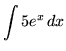. By formula 1 from the introduction to this section on integrating exponential functions and properties of integrals we get that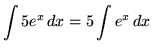.

SOLUTION 2 : Integrate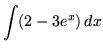. By formula 1 from the introduction to this section on integrating exponential functions and properties of integrals we get that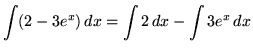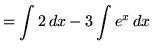.

SOLUTION 3 : Integrate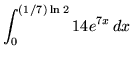. Use u-substitution. Let

u = 7x

so that

du = 7 dx ,

or

(1/7) du = dx .

In addition, the range of x-values is,

so that the range of u-values is,

or.

Substitute into the original problem, replacing all forms of x, getting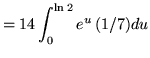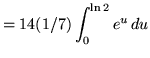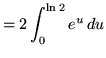(Recall that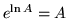.)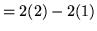= 4 - 2

= 2 .

SOLUTION 4 : Integrate. Use u-substitution. Let

u = 2x+3

so that

du = 2 dx ,

or

(1/2) du = dx .

Substitute into the original problem, replacing all forms of x, getting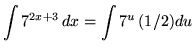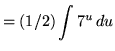(Now use formula 2 from the introduction to this section on integrating exponential functions.)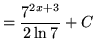(Recall that.)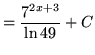.

SOLUTION 5 : Integrate. First, multiply the exponential functions together. The result is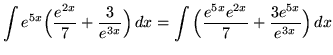(Recall thatand.)(Use the properties of integrals.)(Use formula 3 from the introduction to this section on integrating exponential functions.).